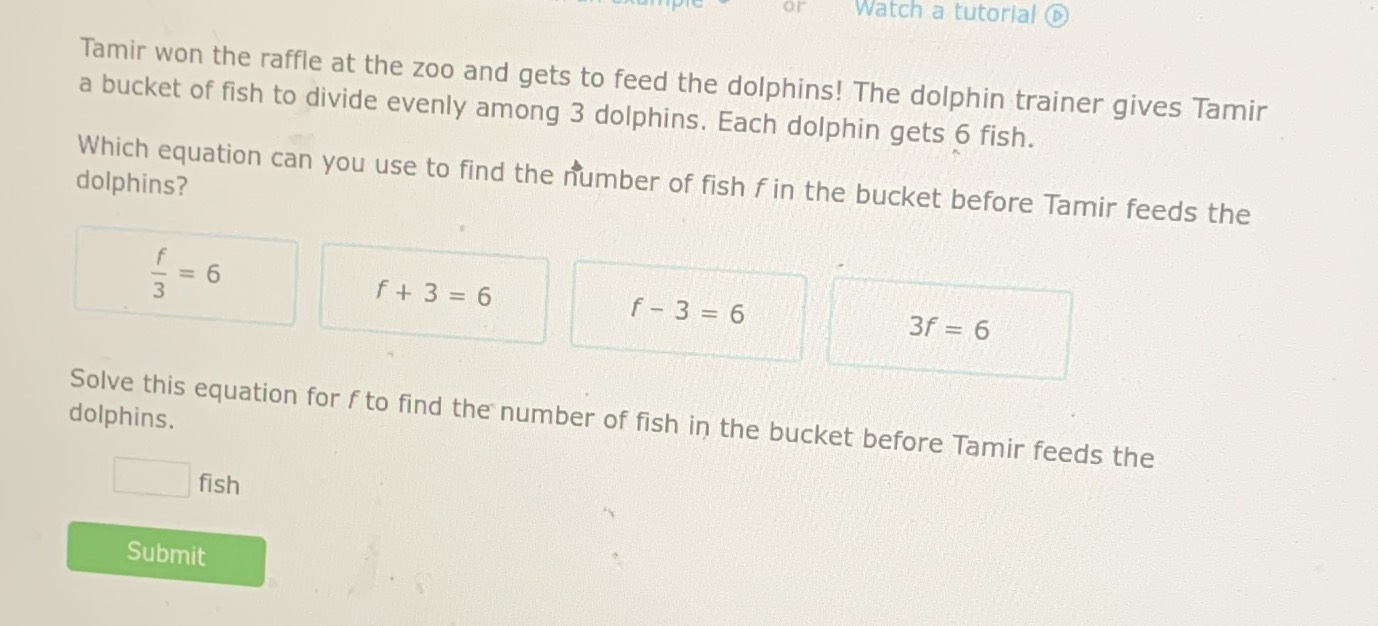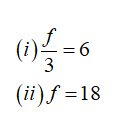### Still have math questions?

Algebra
QuestionTamir won the raffle at the zoo and gets to feed the dolphins! The dolphin trainer gives Tamir a bucket of fish to divide evenly among $$3$$ dolphins. Each dolphin gets $$6$$ fish. dolphins? $$\frac { f } { 3 } = 6 \quad f + 3 = 6 \quad 3 f = 6$$

Solve this equation for $$f$$ to find the number of fish in the bucket before Tamir feeds the dolphins.

fish